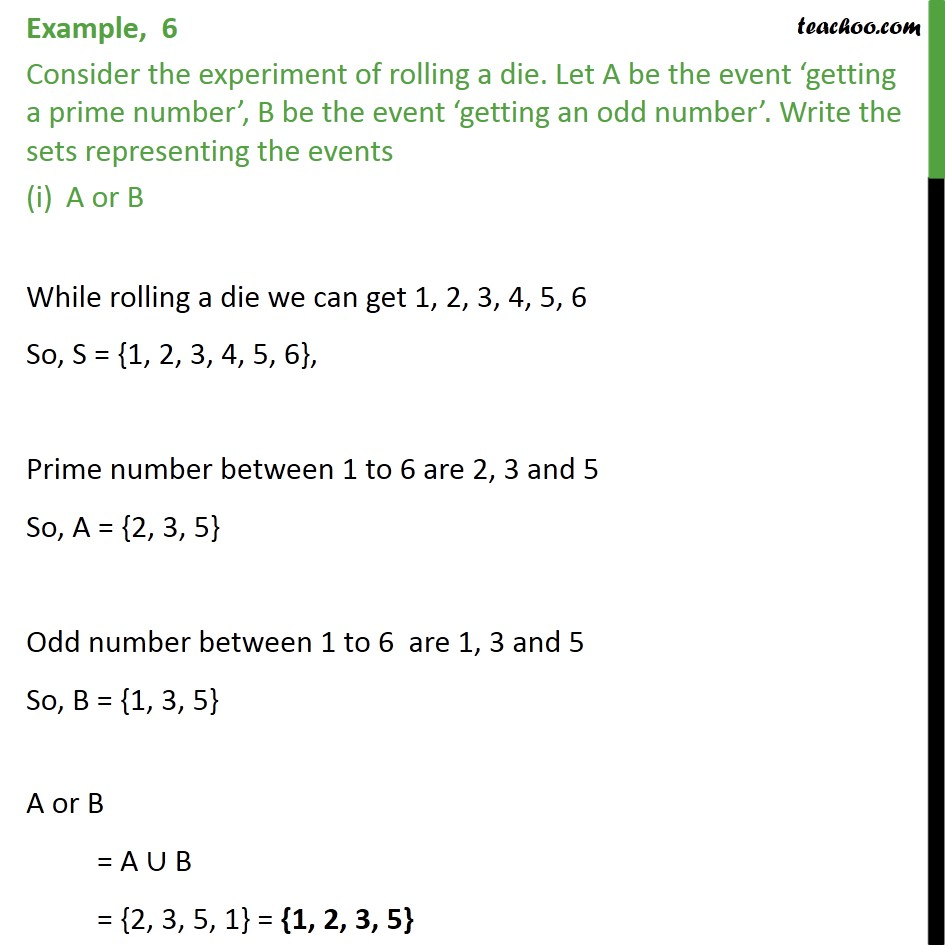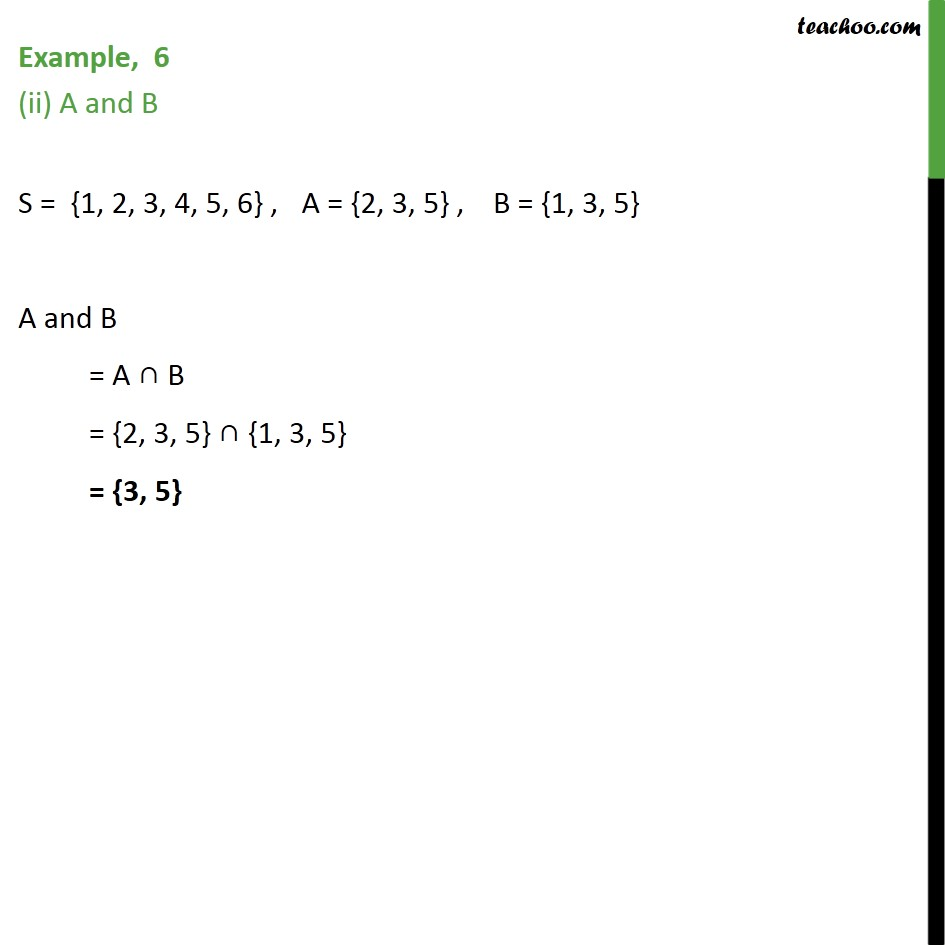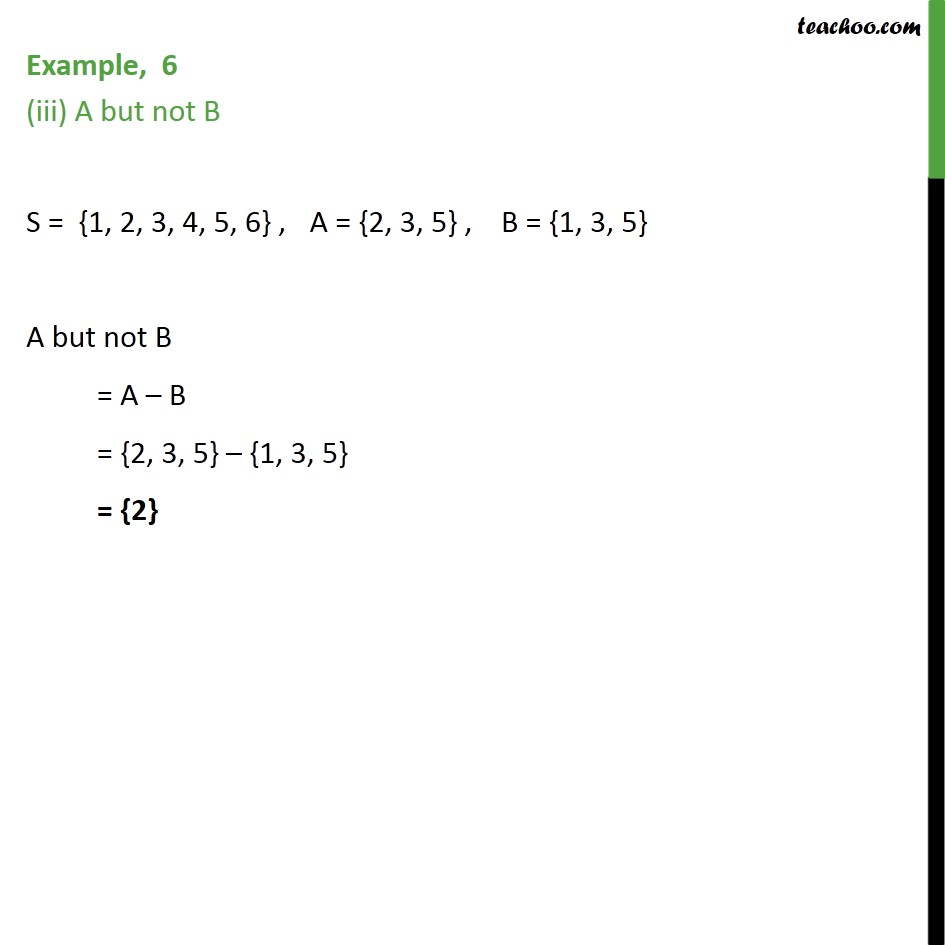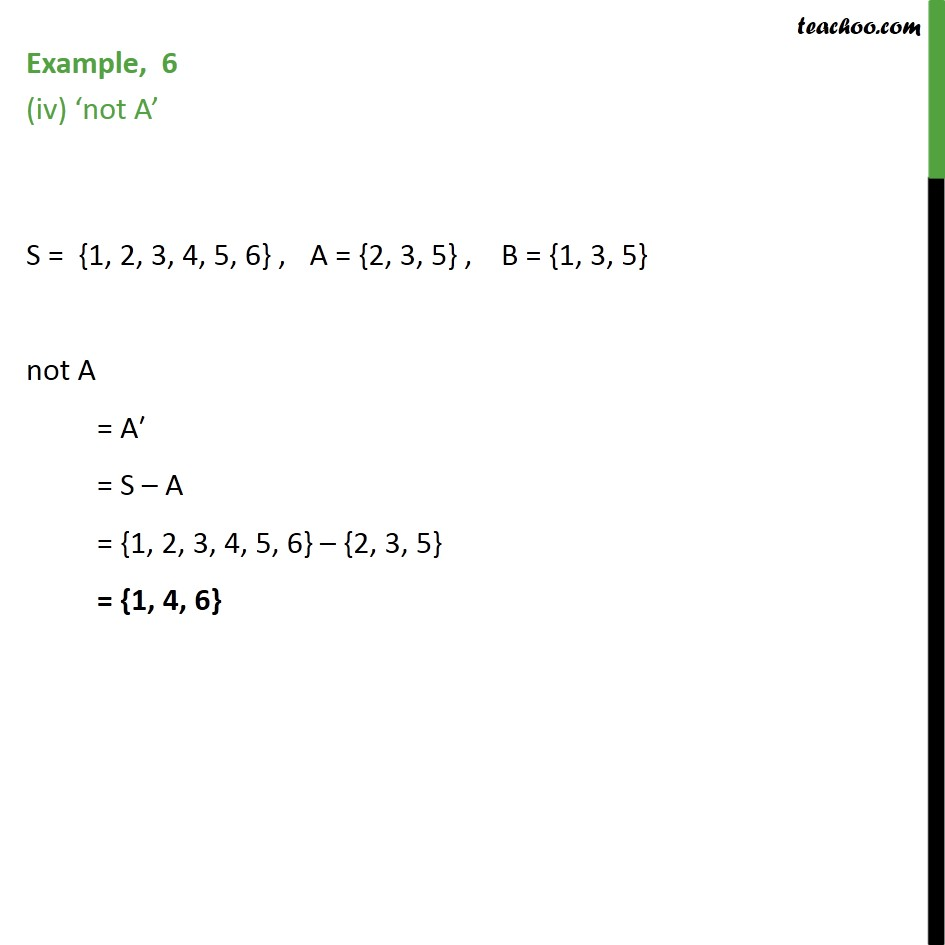Algebra of events

Chapter 16 Class 11 Probability
Concept wiseIntroducing your new favourite teacher - Teachoo Black, at only ₹83 per month

### Transcript

Example, 6 Consider the experiment of rolling a die. Let A be the event ‘getting a prime number’, B be the event ‘getting an odd number’. Write the sets representing the events A or B While rolling a die we can get 1, 2, 3, 4, 5, 6 So, S = {1, 2, 3, 4, 5, 6}, Prime number between 1 to 6 are 2, 3 and 5 So, A = {2, 3, 5} Odd number between 1 to 6 are 1, 3 and 5 So, B = {1, 3, 5} A or B = A ∪ B = {2, 3, 5, 1} = {1, 2, 3, 5} Example, 6 (ii) A and B S = {1, 2, 3, 4, 5, 6} , A = {2, 3, 5} , B = {1, 3, 5} A and B = A ∩ B = {2, 3, 5} ∩ {1, 3, 5} = {3, 5} Example, 6 (iii) A but not B S = {1, 2, 3, 4, 5, 6} , A = {2, 3, 5} , B = {1, 3, 5} A but not B = A – B = {2, 3, 5} – {1, 3, 5} = {2} Example, 6 (iv) ‘not A’ S = {1, 2, 3, 4, 5, 6} , A = {2, 3, 5} , B = {1, 3, 5} not A = A′ = S – A = {1, 2, 3, 4, 5, 6} – {2, 3, 5} = {1, 4, 6}